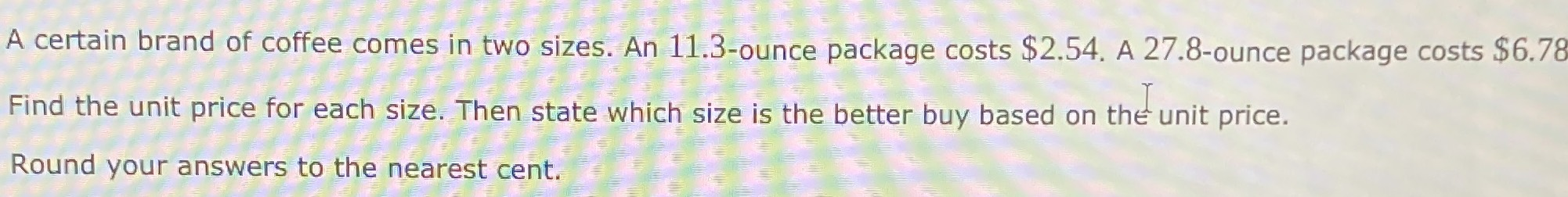### ¿Todavía tienes preguntas de matemáticas?

Pregunte a nuestros tutores expertos
Algebra
PreguntaA certain brand of coffee comes in two sizes. An $$11.3$$ -ounce package costs $$\ 2.54$$ . A 27.8-ounce package costs $$\ 6.78$$. Find the unit price for each size. Then state which size is the better buy based on the unit price. Round your answers to the nearest cent.

unit price of 11.3 ounce package=$0.22 per ounce unit price of 27.8 ounce package=$ 0.24 per ounce